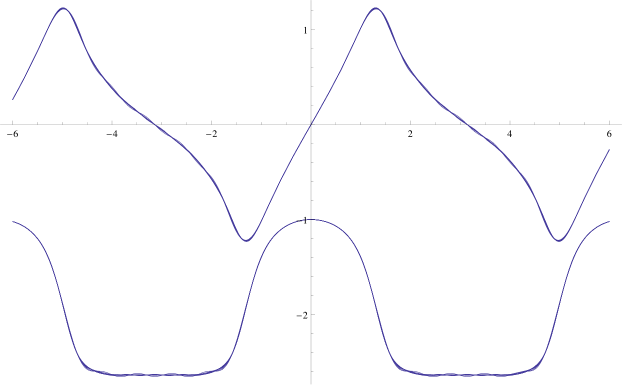# Tetration Forum

Full Version: Super-logarithm on the imaginary line
You're currently viewing a stripped down version of our content. View the full version with proper formatting.
I just found a way to calculate slog on the imaginary axis!
It depends very much on Jay's observation that slog is imaginary-periodic.

Let . S is periodic with period , because . Since S is periodic, we can use Fourier series to represent it. Let , then . The Taylor series coefficients of R will then be the Fourier series coefficients of S. In terms of the super-logarithm, . This means the Fourier series coefficients of are the Taylor series coefficients of which we already know. In other words, , so:

The nice thing about this is that it seems to bypass the radius of convergence problem near z=i since its a Fourier series and not a Taylor series. Is this right?

I've included a plot with multiple approximations, which seem to converge much faster than doing analytic continuation section-by-section. The top line is the imaginary part, and the bottom line is the real part, and the "y" axis is :Andrew Robbins
Actually, if you think about it, we're still limited by the same radius of convergence, because it's the same series.

HOWEVER, because we're plugging e^z into the series, rather than z, we're limited by abs(e^z) < 1.37445, not abs(z)<1.37445.

This essentially means that for all complex values with real part less than 0.31813 (real part of primary fixed point), the series will converge.

So if you plug e^(iz) into the series, and use only real z, then (because the imaginary part is 0, and hence the real part of iz is 0) you will always be inside the radius of convergence. Effectively, you're calculating points on the unit circle with the original series.
By the way, now that I see it written down, I see that I made a simple but important mistake a few days ago, by forgetting the i in the exponents for the exponential form of the fourier series. I knew that we had real exponents for slog, but didn't quite link it up with the fact that we're periodic in the imaginary direction.
Right, since the real part of all points in the "backbone" of the slog are less than the logarithm of the radius of convergence, the exponential of them is within the radius of convergence (of the series expansion about z=0).

Andrew Robbins